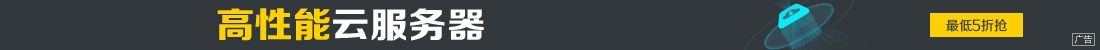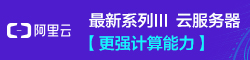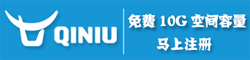# 几种实用的pythonic语法实例代码

••python 是一门简单而优雅的语言，可能是过于简单了，不用花太多时间学习就能使用，其实 python 里面还有一些很好的特性，能大大简化你代码的逻辑，提高代码的可读性。

Beautiful is better than ugly.
Explicit is better than implicit.
Simple is better than complex.
Complex is better than complicated.
Flat is better than nested.
Sparse is better than dense.
Special cases aren't special enough to break the rules.
Although practicality beats purity.
Errors should never pass silently.
Unless explicitly silenced.
In the face of ambiguity, refuse the temptation to guess.
There should be one?C and preferably only one ?Cobvious way to do it.
Although that way may not be obvious at first unless you're Dutch.
Now is better than never.
Although never is often better than *right* now.
If the implementation is hard to explain, it's a bad idea.
If the implementation is easy to explain, it may be a good idea.
Namespaces are one honking great idea ?C let's do more of those!

```def fibonacci():
num0 = 0
num1 = 1
for i in range(10):
num2 = num0 + num1
yield num2
num0 = num1
num1 = num2
for i in fibonacci():
print(i)```

if / else 大家都用过，但是在 python 里面，else 还可以用在循环和异常里面

```# pythonic 写法
for cc in ['UK', 'ID', 'JP', 'US']:
if cc == 'CN':
break
else:
print('no CN')
# 一般写法
no_cn = True
for cc in ['UK', 'ID', 'JP', 'US']:
if cc == 'CN':
no_cn = False
break
if no_cn:
print('no CN')```

else 放在循环里面的含义是，如果循环全部遍历完成，没有执行 break，则执行 else 子句

```# pythonic 写法
try:
db.execute('UPDATE table SET xx=xx WHERE yy=yy')
except DBError:
db.rollback()
else:
db.commit()
# 一般写法
has_error = False
try:
db.execute('UPDATE table SET xx=xx WHERE yy=yy')
except DBError:
db.rollback()
has_error = True
if not has_error:
db.commit()```

else 放到异常里面可以表示，如果没有异常发生需要执行的操作

```# pythonic 写法
with open('pythonic.py') as fp:
for line in fp:
print(line[:-1])
# 一般写法
fp = open('pythonic.py')
for line in fp:
print(line[:-1])
fp.close()```

• 调用 `open('pythonic.py')` ，返回的一个对象 obj,
• 调用 `obj.__enter__() `方法，返回的值赋给 fp
• 执行 with 中的代码块
• 执行 `obj.__exit__()`
• 如果这个过程发生异常，将异常传给 `obj.__exit__() `，如果 `obj.__exit__() `返回 False, 异常将被继续抛出，如果返回 True，异常被挂起，程序继续运行

[expr for iter_var in iterable if cond_expr]

(expr for iter_var in iterable if cond_expr)

```# pythonic 写法
squares = [x * x for x in range(10)]
# 一般写法
squares = []
for x in range(10):
squares.append(x * x)```

python 里面 map 的遍历有很多种方式，在需要同事使用 key 和 value 的场合，建议使用 items() 函数

```m = {'one': 1, 'two': 2, 'three': 3}
for k, v in m.items():
print(k, v)
for k, v in sorted(m.items()):
print(k, v)```

《编写高质量代码：改善 Python 程序的91个建议》